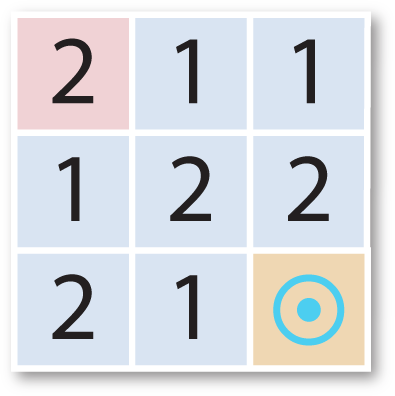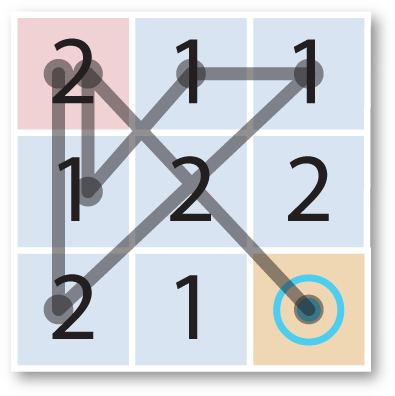## Maximum number of duplicates in a list

23rd August 2019

What's the most efficient way to find the maximum number of duplicates in a list of numbers? The answer is - it depends.

### The application

Here's the motivation I had for solving this problem for a maze puzzle game I was writing. If you want you can skip this section and go straight to the algorithms in the next section.

Here's an example of one of the mazes. In this maze you start in the top left corner, and you have to find the shortest route to the goal in the bottom right corner. The number on your current cell tells you how far you can jump. You start moving horizontally/vertically, your next jump is diagonal, and so on, alternating between horizontal/vertical and diagonal on every move:Here's the solution:Numbering the cells from 0 in the top left corner to 8 in the bottom right corner this solution can be represented by the list:

`(0 6 2 1 3 0 8)`

This is a simple example of a two-state maze; you may have to revisit one or more cells twice to solve the maze; in this case cell 0 is visited twice.

### The function

I wanted to write a routine max-duplicates which takes a list containing n integers, each of which can be in the range 0 to n-1, and finds the largest number of duplicates in the list; so:

`(max-duplicates '(0 6 2 1 3 0 8))`

should return 2.

An initial attempt

The most obvious way to solve this is to scan through the list, counting the number of occurrences of each integer in a separate array of counts. Here's the function:

```(defun max-duplicates1 (list)
(let ((a (make-array (length list) :initial-element 0))
(count 0) best))
(map nil #'(lambda (n) (incf (aref a n))) list)
(reduce #'max a))```

This has the advantage of only needing one pass through the list, but requires the additional storage of an array of integers.

Avoiding storage

It seemed wasteful to have to create an array of counts in max-duplicates1, so I thought of a different approach. We can avoid the array of counts by sorting the list into ascending order; we can then just make one pass through the list to find the longest sequence of identical integers. Here's the routine:

```(defun max-duplicates2 (list)
(let* ((sorted (sort (copy-list list) #'<))
(item (car sorted))
(count 0) (best 0))
(map nil #'(lambda (x)
(cond
((= item x) (incf count))
(t (when (> count best) (setq best count))
(setq count 1)
(setq item x))))
sorted)
(max count best)))```

A third attempt

The problem with max-duplicates2 is that we have to sort a long list. Most sorting algorithms have an efficiency of either O(n^2) or O(n*log(n)), which is going to be a significant penalty with a long list.

I therefore tried a modified version of the first approach, reducing the amount of storage needed by keeping a bit array to record whether we have found one occurrence of an integer in the list. We then maintain an association list of (number . count) for integers that occur more than once.

Here's the routine:

```(defun max-duplicates3 (list)
(let* ((len (length list))
(a (make-array len :element-type 'bit :initial-element 0))
(best nil) (max 0))
(map nil #'(lambda (n)
(cond
((= (aref a n) 1)
(let ((item (assoc n best)))
(cond
(item (incf (cdr item)))
(t (push (cons n 2) best)))))
(t (incf (aref a n)))))
list)
(reduce #'max best :key #'cdr)))```

### Comparing the routines

To compare these routines I created a list of 100000 random numbers:

```(defparameter *test*
(map 'list #'(lambda (x) (declare (ignore x)) (random 100000))
(make-list 100000)))```

This random list typically has a maximum of 8 duplicates. Here's how they compare with this random list:

 Time (secs) Storage (bytes) max-duplicates1 0.022 492856 max-duplicates2 0.115 1296384 max-duplicates3 2.150 754644

So I could have saved my time and gone with the first simple-minded approach, and the third approach is really poor.

But wait a minute: what about a list in which the probability of a duplicate is very small?

Here's a routine to create a second test list of 100000 numbers. First I set the integer in position n to n, and then randomly swap pairs of numbers, using the handy Common Lisp swap function rotatef. Finally I make one duplicate somewhere in the list:

```(defparameter *test*
(let ((a (make-array 100000)))
(dotimes (x 100000) (setf (aref a x) x))
(dotimes (x 100000)
(rotatef (aref a x) (aref a (random 100000))))
(setf (aref a (random 100000)) (aref a (random 100000)))
(coerce a 'list)))```

Here are the timings for this second list, which has only 2 identical integers:

 Time (secs) Storage (bytes) max-duplicates1 0.019 497288 max-duplicates2 0.113 1297132 max-duplicates3 0.002 66412

The performance of the first two approaches is approximately the same, but now my third routine, max-duplicates3, is far more efficient than either.

So the moral of this story is: the most efficient routine may depend not only on the size of your input, but also how disorganised it is.